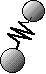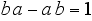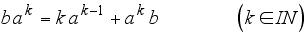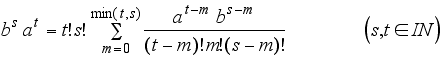back to oscillator page

###Sketch: Energy Spectrum of the Quantum Theoretical Oscillator

Symbols like "a" or "b" can be concatenated or juxtaposed, giving the prototype of a multiplication. Disrespecting parenthization (ab)a=a(ba)=aba is called associativity of the multiplication. Respecting the order of the symbols ab and ba makes the multiplication noncommutative. (In mathematics the emerging structure is called a free monoid generated by the elements "a" and "b". )

The monoid elements can be written as being added by grouping them around a "plus"-sign. And a monoid element can be written as being multiplied by a number (complex or real) by prefixing it with the given number.  This is the basis for realizing the Heisenberg commutation-relation with monoids (The one represents the neutral element of the monoid-multiplication.):Iterations over the natural numbers give:And the energy eigenvalue problem of the one-dimensional harmonic oscillator then can be formulated as follows:That problem is solved by using extensions of linear combinations to infinitely many summands (giving the notion of the so-called "reduced basis" of a Hausdorff topological vector space as a counterpart to the commonly used algebraic basis of a vector space (Furthermore, the reduced basis is something fundamentally different than a topological basis of a vector space.)). The eigenvalues are as to be expected:This constitutes a new method of solving the energy-eigenproblem of the harmonic oscillator in quantum theory.

###back to oscillator page